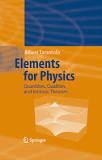## Monday, September 7, 2015

### Elements for Physics Quantities, Qualities, and Intrinsic Theories by Albert TarantolaWhile usual presentations of physical theories emphasize the notion of physical quantity, this book shows that there is much to gain when introducing the notion of physical quality. The usual physical quantities simply appear as coordinates over the manifolds representing the physical qualities. This allows to develop physical theories that have a degree of invariance much deeper than the usual one. It is shown that properly developed physical theories contain logarithms and exponentials of tensors: their conspicuous absence in usual theories suggests, in fact, that the fundamental invariance principle stated in this book is lacking in present-day mathematical physics. The book reviews and extends the theory if Lie groups, develops differential geometry, proposing compact definitions of torsion and of curvature, and adapts the usual notion of linear tangent application to the intrinsic point of view proposed for physics. As an illustration, two simple theories are studied with some detail, the theory of heat conduction and the theory of linear elastic media. The equations found differ quantitatively and qualitatively from those usually presented.